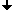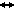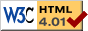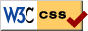Nonlinear Dynamics at the Free University Berlin

Summer 2012

# Differential Equations I

PD Dr. Pavel Gurevich

Recitation session: Dr Sergey Tikhomirov, Eyal Ron

## Schedule, Summer 2012

Lecture:
Tuesday 10.15-12.00, Takustraße 9 SR 049
Thursday 10.15-12:00, Arnimallee 6 SR 025/026
Recitation session:
Friday, 10.15-12.00 (Eyal Ron),Arnimallee 6 SR 007/008
Friday, 12.15-14.00 (Sergey Tikhomirov), Arnimallee 3, Room 119

## Topics

In this course, one gets to know what ordinary differential equations are, how they naturally arise, how one can try to solve them. It is however often the case that one cannot explicitly solve a differential equation. It is therefore important to know whether a solution still exists, which properties it possesses (even without having explicit formulas for a solution), whether it makes sense to solve it numerically, etc. The following classical topics will be covered in the course:

1. General notions of differential equation. Motivating examples.
2. Existence theory.
3. Continuous dependence of solutions on initial data.
4. Solving some elementary differential equations.
5. Linear differential equations with constant coefficients.
6. Linear differential equations with variable coefficients.
7. Nonlinear autonomous differential equations: phase spaces, flows.
8. Nonlinear autonomous differential equations: stationary points, stability.
9. Stable and unstable manifolds.
10. Periodic solutions of differential equations and their stability.

## Literature

1. H. Amann. Ordinary differential equations. Walter de Gruyter, 1990.
2. L.S. Pontryagin. Ordinary Differential Equations. Addison-Wesley Pub. Co., 1962.
3. V.I. Arnold. Ordinary Differential Equations. Springer, 1992.
4. P. Hartman. Ordinary Differential Equations. Cambridge University Press, 2002.

## Homework assignments, Summer 2012

1. First assignment, due Friday ,April 20, 2012, at 10:00, (PDF)
2. Second assignment, due Friday ,April 27, 2012, at 10:00, (PDF)
3. Third assignment, due Wednesday ,May 02, 2012, at 13:00, (PDF)
4. Forth assignment, due Wednesday ,May 09, 2012, at 13:00, (PDF)
5. Fifth assignment, due Wednesday ,May 16, 2012, at 13:00, (PDF)
6. Sixth assignment, due Wednesday ,May 23, 2012, at 13:00, (PDF)
7. Seventh assignment, due Wednesday ,May 30, 2012, at 13:00, (PDF)
Corrected version of problem 25. New deadline for this problem: Wednesday, June 06, 2012, at 13:00 (PDF)
8. Eighth assignment, due Wednesday ,June 06, 2012, at 13:00, (PDF)
9. Ninth assignment, due Wednesday ,June 13, 2012, at 13:00, (PDF)
10. Tenth assignment, due Wednesday ,June 20, 2012, at 13:00, (PDF)
Corrected version of problem 33. (PDF)
11. Eleventh assignment, due Wednesday ,June 27, 2012, at 13:00, (PDF)
12. Twelfth assignment, due Wednesday ,July 4, 2012, at 13:00, (PDF)
13. Additional Problems for grade improvement, due Wednesday ,July 6, 2012, at the tutorial, (PDF)

Please use the appropriate boxes, Arnimallee 3, upstairs.

## Exam

The written exam will take place on 12.07.2012, 10:00-12:00.
Sample exam (PDF).
Topics for the exam on Differential Equations I (PDF).
Equations to practice (PDF), answers (PDF).
Exam Result (docx) (pdf).

## Second exam

The second written exam will take place on October 11, 2012, 14:00-16:00 at Arnimallee 6 SR 025/026.Last change: Oct. 7, 2012
 This page strictly conforms to the HTML4.01 standard and uses style sheets.